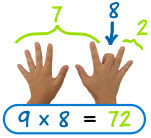## Search Here

### SIMPLE MATHEMATICS TRICS - MULTPLICATION

Multiply
by
Trick
2
add the number to itself (example 2×9 = 9+9)
5
the last digit goes 5, 0, 5, 0, ...

is always half of 10× (Example: 5x6 = half of 10x6 = half of 60 = 30)

is half the number times 10 (Example: 5x6 = 10x3 = 30)
6
when you multiply 6 by an even number, they both end in the same digit.
Example: 6×2=12, 6×4=24, 6×6=36, etc
9the last digit goes 9, 8, 7, 6, ...
your hands can help! Example: to multiply 9 by 8, hold your 8th finger down, and count "7" and "2", the answer is 72
is 10× the number minus the number. Example: 9×6 = 10×66 = 60−6 = 54

when you add the answer's digits together, you get 9.
Example: 9×5=45 and 4+5=9. (But not with 9×11=99)
10
put a zero after it
11
up to 9x11: just repeat the digit (Example: 4x11 = 44)
for 10x11 to 18x11: write the sum of the digits between the digits
Example: 15x11 = 1(1+5)5 = 165

Note: this works for any two-digit number, but when the sum of the digits is more than 9, we need to"carry the one". Example: 75x11 = 7(7+5)5 = 7(12)5 = 825.
12
is 10× plus 2×

Key Words : Nobel Prize 2014, Kerala PSC, Secretariat Assistant